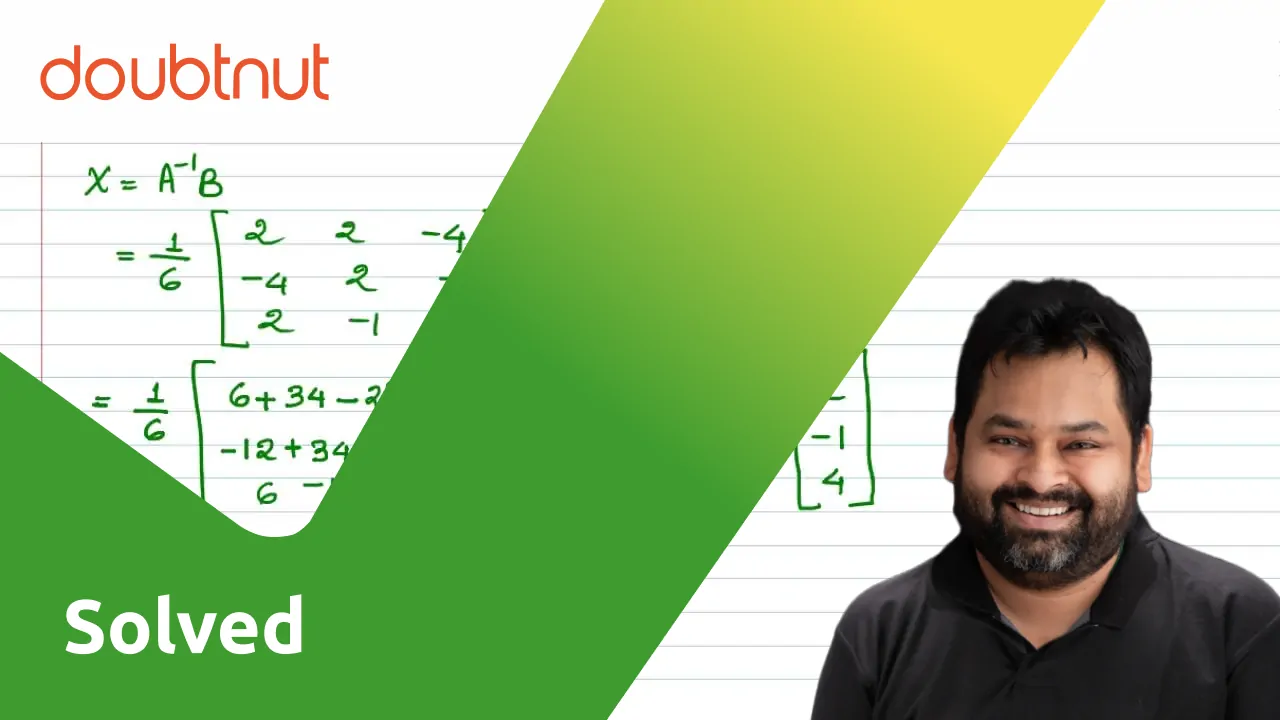Doubtnut App - Get 50 Lakh+ video solutions better than GoogleInstall Now

# Given that A=⎡⎢⎣1−10234012⎤⎥⎦ and B=⎡⎢⎣22−4−42−42−15⎤⎥⎦. Find AB. Use this to solve that following system of equations : x−y=3, 2x+3y+4z=17, y+2z=7.

Text Solution
Verified by Experts

## The correct Answer is:AB=6I; x=2, y=−1, z=4

Video Solution